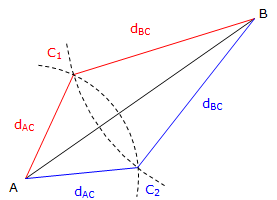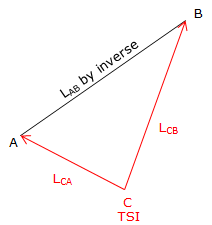2. Types of intersections

The three primary types of intersection are:

• Distance-Distance
• Angle-Distance
• Angle-Angle

The intersection name identifies the two additional measurements made to solve the triangle. Most other types of intersections are a variation of one of these three primary ones

In the following discussions points A and B are control points with known coordinates and therefore provide a known side (base line) of a triangle by inversing. Point C is the one whose coordinates are to be determined. A basic solution is outlined for each intersection method.

To minimize repetition, each method starts out first with an inverse computation to determine the length, LAB, and direction, DirAB (or DirBA) of the base line.

a. Distance-Distance

Two distances are measured to Point C, one from each end of the base line. This gives us lengths of three triangle sides.Figure D-2 Distance-Distance Intersection

However, there is more than a single solution for point C, Figure D-3.Figure D-3 Multiple Solutions

Triangles A-B-C1 and A-B-C2 are identical mirror images - they have the same angle and distance values. It's up to the surveyor to decide which point C is to be solved (this is the primary reason we always use at least three distances in tie notes).

A single intersection point would only occur if dAB = dAC+dBC in which case point C would be on the base line.

To solve point C:

Use the Law of Cosines to solve for angle at B.

Use DirBA and angle B to determine the direction of BC. This must be done by inspection based on the appropriate point C: for C1 add angle B to DirBA; for C2 subtract angle B from DirBA.

Perform a forward computation from B using the length and direction of BC.

Math Check:

Use the Law of Cosines to solve for angle at A.

Use DirAB and angle A to determine the direction of AC. This must be done by inspection based on the appropriate point C: for C1 subtract angle A from DirAB; for C2 add angle A to DirAB

Perform a forward computation from A using the length and direction of AC.

Both coordinate sets for C should be the same, allowing for rounding errors.

b. Angle-Distance Intersection

A distance is measured from one end of the base line; an angle is measured from the other. This results in a triangle for which two sides and one angle are known.Figure D-4 Multiple Solutions

Since a distance is involved, there are two possible locations for point C as shown in Figure D-4. A single intersection would occur only if the angle at C were exactly 90°.

To solve:

Start by using the Law of Sines to solve for the angle at C. The Law of Sines will return the angle C2 which is the one less than 90°. Remember that sin(C1) = sin(C2) so that C1 = 180° - C2. It's up to the surveyor to decide which of the two angles fits the situation.

Once that's resolved, use Law of Sines to compute the distance from B to C.

Use DirBA and the angle at B to compute the direction from B to C.

Perform a forward computation from B using the length and direction of BC.

Math Check:

Use angle B and angle C from the previous solution to solve for the angle at A.

Use DirAB and angle A to determine the direction of AC.

Perform a forward computation from A using the length and direction of AC.

Both coordinate sets for C should be the same, allowing for rounding errors.

c. Angle-Angle Intersection

The angles at both ends of the base line are measured to the unknown point. From these angles directions to the point can be computed.Figure D-5 Angle-Angle Intersection

Two directions can only intersect at a single point so there are no multiple solutions for this method as with the others.

To solve point C's position:

Use angles at A and B to solve for angle at C.

Use the Law of Sines to solve the distance AC.

Use DirAB and angle A to compute DirAC.

Perform a forward computation from A using the length and direction of AC.

Math Check:

Use angle C from above and the Law of Sines to solve the distance from B to C.

Use DirBA and angle B to compute DirBC.

Perform a forward computation from B using the length and direction of BC.

Both coordinate sets for C should be the same, allowing for rounding errors.

d. Other intersections

Other intersection types are generally variations of the three primary intersections. Some examples are:

(1) Direction-Distance and Direction-Direction

These are vesions of Angle-Distance and Angle-Angle intersections. The difference is that an angle is referenced to the base line while a direction is referenced to a meridian. In the course of solving an Angle-Distance or Angle-Angle intersection, line directions are computed so Direction-Distance and Direction-Direction are intermediate steps.(a) Direction-Distance (b) Direction-Direction Figure D-6 Intersection Variations

(2) Circle-Circle (a.k.a. Arc-Arc)

 Two arc radii are intersected to locate the unknown position. Base line end points are the radius points. Since radii are distances, this is the same as a Distance-Distance intersection, with the same multiple position situation for the unknown point.Figure D-7 Circle-Cirvle Intersection

(3) Circle-Angle (Arc-Angle) intersectionThis is the same as an Angle-Distance intersection. One end of the base line serves as the radius point from which the distance is measured. This method also results in two possible locations for the unknown point. Figure D-8 Circle-Angle Intersection

(4) Right Angle Offset

 At one end of the base line, a 90° angle is turned and a distance to the unknown point is measured. The coordinates of the unknown point are computed using a forward computation from the measurement location.Figure D-9 Right Angle Offset

e. Field Modifications

In all the methods discussed (except Right Angle Offset), angle and/or distance measurements were made from both ends of the base line. In order to turn an angle from one end of the base line, the other end must be visible to serve as a back sight. In the field, this isn't always practical or efficient. The key to intersection solution is that the unknown point must be visible from both ends of the base line, or, conversely, the end points are visible from the unknown point. This allows the surveyor to make a single instrument set up - at the unknown point.

 The base line still forms a known side of the triangle even if the end points are not intervisible. That means two other measurements are necessary in order to fix the triangle. With a total station instrument (TSI) set up at the unknown point, what measurements should be made?Figure D-10 Total Station LocationMeasure distances to the base line end points. Begin solving by using Law of Cosines. Figure D-11 Location by DistancesMeasure distance to one end of the base line and the angle between the endpoints. Begin solving by using Law of Sines Figure D-12 Location By Distance and AngleMeasure distance to other end of the base line and the angle between the end points. Begin solving by using Law of Sines. Figure D-13 Location By Angle and Other DistanceTo provide an independent check, measure both distances and the angle. Figure D-14 Two Distances and One Angle